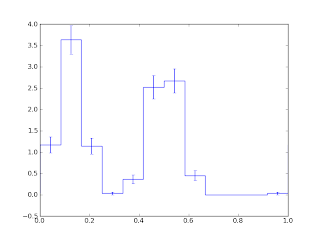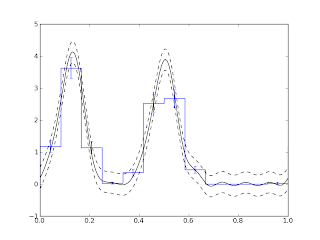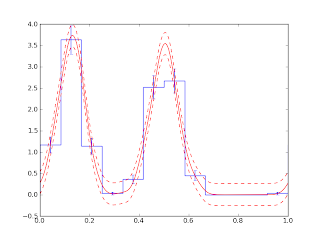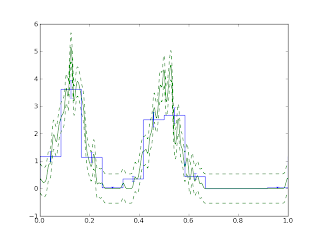### Kernel Density Estimators

Since high school science class, I've been making graphs that show one variable as a function of another - force as a function of extension, redshift as a function of distance, intensity as a function of wavelength, et cetera. But around that time I was also writing code - probably generating some kind of chaos or fractal - that needed to plot the distribution of data points in one dimension. Seems simple, right? Well, the standard solution is to produce a histogram - divide up the range into "bins", count how many fall into each bin, and plot that. But choosing the right number of bins is something of an art: too many and your plot is hopelessly spiky, too few and you can't see any features your data might have. So I wondered if there was something better. It turns out that there is (though it's not always clear when it actually is better); a tool called a kernel density estimator.First, the problems with histograms. I generated a collection of 400(*) photon arrival phases corresponding to two equal peaks. Above is plotted a histogram of their arrival times. Below is a histogram of their arrival times shifted by half a bin. To me, at least, it's not at all obvious that the two histograms are of the same distribution, let alone the same sample.Since the signal is periodic, one natural thing to try is to work with the Fourier series. It's not too hard to construct some coefficients of the Fourier series of the photon arrival times. If I used all (infinitely many) coefficients I'd get a collection of spikes, one at each photon arrival time, which isn't too useful. But if I discard all Fourier coefficients after a certain point, I smooth out those spikes into a more reasonable shape. Here's what I get using ten harmonics:The two peaks look more similar now, which makes sense since the Fourier coefficients don't really care about the starting phase. There are those nasty ripples, though - in fact, they go negative, which is rather distressing for something that's supposed to be a probability distribution. (Incidentally, there's some Fourier magic that goes into that error band on the sinusoid but I don't want to get into it right now.)

One way to get rid of those ripples is to think of this as a problem in digital filtering, where they are an example of the "ringing" that can occur in digital filters with too-sharp cutoffs. In that context, the usual solution is to taper the coefficients to zero smoothly, and that's what I will do. But there is a statistical way to think about what's going on.

First of all, tapering or cutting off the Fourier coefficients can be thought of as multiplying the Fourier coefficients by another set of Fourier coefficients. By a wonderful theorem of Fourier analysis, this amounts to convolving the set of photon arrival times by a kernel. That is, we take the forest of delta-functions representing the photon arrival times, and replace each delta function with a copy of some smoother function, and add them all together. This process, when carried out on the real line, is known to statisticians as constructing a kernel density estimator (the "kernel" is the smoother function, and it is used to estimate a probability density).

Simply chopping off the Fourier coefficients corresponds to the kernel sin((n+1/2)x)/sin(x/2), the periodic "sinc" function. This has a peak at zero but oscillates from positive to negative, so it's not too surprising that we got ripples. So to get rid of the ripples, we just choose a kernel that is positive and whose Fourier coefficients we know. There is a natural (if somewhat obscure) candidate: the von Mises probability distribution. This is a circular analog of a Gaussian, both in appearance and in a technical sense: the Gaussian distribution is the distribution with maximum entropy for specified mean and standard deviation. The von Mises distribution has maximum entropy for specified "circular mean" (which includes information about spread as well as location). In any case, it's a positive smooth periodic distribution whose Fourier coefficients can be computed in terms of Bessel functions using your favourite scientific computing tool. So using it instead gives this:This looks pretty good; it shows the distribution pretty cleanly, with no ringing. There's only one fly in the ointment: while I don't need to specify a starting phase, I do need to come up with a parameter - analogous to the number of harmonics - that determines the width of the kernel. If I choose too wide a kernel, it flattens out all the features in my data; if I choose too narrow a kernel I get something horrible like this:So in a way I haven't solved the original problem with histograms that motivated me. Fortunately, one of the selling points of kernel density estimators is that the statistical literature is full of papers on how programs can automatically choose the degree of smoothing. None of their techniques (that I have found) are appropriate for periodic data, but I have my own ideas about that (to be written up in future).

(*) 400 is not actually a very small number of photons; Fermi often gets something like one photon a week from a source.